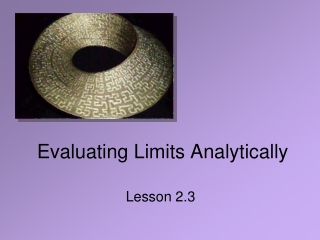Download PresentationEvaluating Limits Analytically

Evaluating Limits Analytically

Télécharger la présentationEvaluating Limits Analytically

- - - - - - - - - - - - - - - - - - - - - - - - - - - E N D - - - - - - - - - - - - - - - - - - - - - - - - - - -
Presentation Transcript

1. Evaluating Limits Analytically Lesson 2.3

2. What Is the Squeeze Theorem? Today we look at various properties of limits, including the Squeeze Theorem

3. Basic Properties and Rules • Constant rule • Limit of x rule • Scalar multiple rule • Sum rule(the limit of a sum is the sum of the limits) See other properties pg. 79-81

4. Limits of Functions • Limit of a polynomial P(x) • Can be demonstrated using the basic properties and rules • Similarly, note the limit of a rational function What stipulation must be made concerning D(x)?

5. Try It Out • Evaluate the limits • Justify steps using properties

6. General Strategies

7. Some Examples • Consider • Why is this difficult? • Strategy: simplify the algebraic fraction

8. Reinforce Your Conclusion • Graph the Function • Trace value close tospecified point • Use a table to evaluateclose to the point inquestion

9. Some Examples • Rationalize the numerator of rational expression with radicals • Note possibilities for piecewise defined functions

10. Three Special Limits • Try it out! View Graph View Graph View Graph

11. Squeeze Rule • Given g(x) ≤ f(x) ≤ h(x) on an open interval containing cAnd … • Then

12. Assignment • Lesson 2.3A • Page 87 • Exercises 1-43 odd • Lesson 2.3B • Page 88 • Exercises 45 – 97 EOO